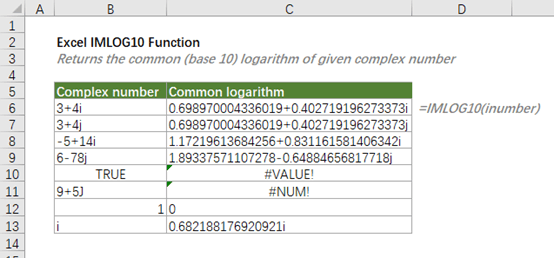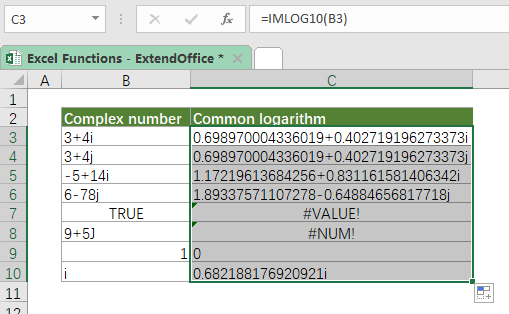## Excel IMLOG10 Function

The IMLOG10 function returns the common (base 10) logarithm of a given complex number in x + yi or x + yj text format.#### Syntax

=IMLOG10(inumber)

#### Arguments

• inumber (required): A complex number for which to get the common (base 10) logarithm .

#### Return Value

The IMLOG10 function returns a complex number as text.

#### Function Notes

• inumber can be supplied as any of the following:
• A real number whose imaginary part is 0, e.g., 1 is a complex number 1+0i; Or a purely imaginary number whose real part is 0, e.g., i is a complex number 0 + 1i;
• A cell reference that refers to a complex number;
• A complex number enclosed in double quotation marks.
• inumber can be returned by the COMPLEX function which converts real and imaginary coefficients into a complex number.
• IMLOG10 will return the #NUM! error if inumber is not recognized as a complex number that has lowercase i or j (iota).
• IMLOG10 will return the #VALUE! error if inumber is a logical value.
• The common (base 10) logarithm of a complex number is:
log10(x + yi) = (log10e)ln(x +yi)
OR
log10(x + yj) = (log10e)ln(x +yj)
where e (Euler's number) is approximately equal to 2.71828.
• To return the logarithm of a number using any base, use the LOG function.

#### Example

To get the common logarithm of the complex numbers listed in the below table, please copy or enter the formula below in the top cell, press Enter to get the result, and then drag the fill handle (at the lower right corner of the result cell) down to apply the formula to below cells.

=IMLOG10(B3)Also, you can type the actual complex number in the formula as shown below. Make sure the complex number is enclosed with double quotes:

=IMLOG10("3+4i")

#### Related functions

Excel COMPLEX Function

The COMPLEX function converts real and imaginary coefficients to a complex number of the form x + yi or x + yj.

Excel IMLN Function

The IMLN function returns the natural logarithm of a given complex number in x + yi or x + yj text format. The natural logarithm is the log value with base e (Euler's number), where e is approximately equal to 2.71828.

Excel IMLOG2 Function

The IMLOG10 function returns the base-2 logarithm of a given complex number in x + yi or x + yj text format.

Excel LOG Function

The LOG function returns the logarithm of a number, using a specified base.

### The Best Office Productivity Tools

#### Kutools for Excel - Helps You To Stand Out From Crowd

 Popular Features: Find, Highlight or Identify Duplicates  |  Delete Blank Rows  |  Combine Columns or Cells without Losing Data  |  Round without Formula ... Super VLookup: Multiple Criteria  |  Multiple Value  |  Across Multi-Sheets  |  Fuzzy Lookup... Adv. Drop-down List: Easy Drop Down List  |  Dependent Drop Down List  |  Multi-select Drop Down List... Column Manager: Add a Specific Number of Columns  |  Move Columns  |  Toggle Visibility Status of Hidden Columns  |  Compare Columns to Select Same & Different Cells ... Featured Features: Grid Focus  |  Design View  |  Big Formula Bar  |  Workbook & Sheet Manager | Resource Library (Auto Text)  |  Date Picker  |  Combine Worksheets  |  Encrypt/Decrypt Cells  |  Send Emails by List  |  Super Filter  |  Special Filter (filter bold/italic/strikethrough...) ... Top 15 Toolsets:  12 Text Tools (Add Text, Remove Characters ...)  |  50+ Chart Types (Gantt Chart ...)  |  40+ Practical Formulas (Calculate age based on birthday ...)  |  19 Insertion Tools (Insert QR Code, Insert Picture from Path ...)  |  12 Conversion Tools (Numbers to Words, Currency Conversion ...)  |  7 Merge & Split Tools (Advanced Combine Rows, Split Excel Cells ...)  |  ... and more

Kutools for Excel Boasts Over 300 Features, Ensuring That What You Need is Just A Click Away...#### Office Tab - Enable Tabbed Reading and Editing in Microsoft Office (include Excel)

• One second to switch between dozens of open documents!
• Reduce hundreds of mouse clicks for you every day, say goodbye to mouse hand.
• Increases your productivity by 50% when viewing and editing multiple documents.
• Brings Efficient Tabs to Office (include Excel), Just Like Chrome, Edge and Firefox.